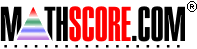Math Practice Online > free > lessons > Florida > 6th grade > Integer Equivalence

## Integer Equivalence

Helps you understand that there really is little difference between a subtraction sign and a negative sign by asking you to create an equivalent expression in a similar fashion to the commutative property.

 Sample Problems for Integer Equivalence Lesson for Integer Equivalence

### This topic aligns to the following state standards

Grade 6: Num 4. Uses concrete models and real-world examples to explore the inverse relationship of positive and negative numbers.
Grade 7: Num 4. Knows the inverse relationship of positive and negative numbers.
Grade 8: Num 2. Knows the inverse relationship of positive and negative numbers.

Copyright Accurate Learning Systems Corporation 2008.